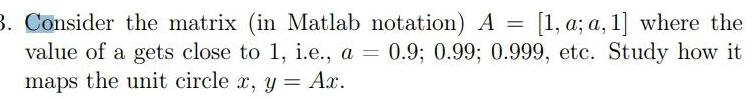Question:

# 3 Consider the matrix in Matlab notation A 1 a a 1 where the

Last updated: 9/10/20233 Consider the matrix in Matlab notation A 1 a a 1 where the value of a gets close to 1 i e a 0 9 0 99 0 999 etc Study how it maps the unit circle x y Ax